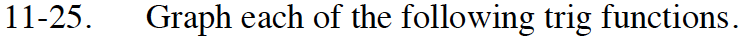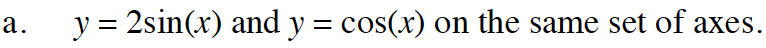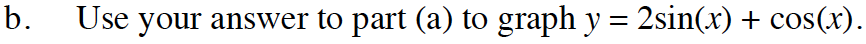### Home > INT3 > Chapter 11 > Lesson 11.1.2 > Problem11-25

11-25.
1. Graph each of the following trig functions. 11-25 HW eTool (Desmos). Desmos Accessibility Homework Help ✎

1. y = 2sin(x) and y = cos(x) on the same set of axes.

2. Use your answer to part (a) to graph y = 2sin(x) + cos(x).Review the Math Notes box in Lesson 9.2.4.$\text{Try adding and then graphing the }y\text{-values of each function for each }\frac{\pi}{2}\text{ multiple of }x.$